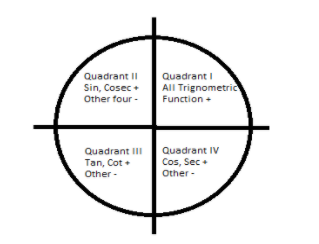QuestionAnswers

# If $\sin {15^ \circ } = \cos \left( {n \times {{15}^ \circ }} \right)$, then n=______.A. 1B. 2C. 5D. 0Hint: When two lines one real axis and the other imaginary axis passes perpendicular through a circle, the circle is divided into four quadrants. Into Quadrant I where both x and y axis are positive, Quadrant II here x axis is negative and y axis is positive, Quadrant III here both the x axis and y axis are negative and in Quadrant IV x-axis is positive and y-axis is negative.
In case of the trigonometric functions in Quadrant I all the functions are positive, in Quadrant II Sin and Cosec functions are positive and other functions are negative, in Quadrant III tan and cot functions are positive and other are negative and in the case of Quadrant IV Cos and Sec functions are positive and other being negative.In this question for the given equation we will cofunction identities of the trigonometric function.

Complete step by step solution: $\sin {15^ \circ } = \cos \left( {n \times {{15}^ \circ }} \right)$
By the rule of cofunction identity,$\sin \theta = \cos \left( {{{90}^ \circ } - \theta } \right)$
We can write as:
$\sin \theta = \cos \left( {{{90}^ \circ } - \theta } \right) \\ \sin {15^ \circ } = \cos \left( {{{90}^ \circ } - {{15}^ \circ }} \right) \\ = \cos \left( {{{75}^ \circ }} \right) \\ \\$
We can also write, $\cos \left( {{{75}^ \circ }} \right) = \cos \left( {5 \times {{15}^ \circ }} \right) = \cos \left( {n \times {{15}^ \circ }} \right)$
Hence, the value of n=5,
Where $\sin {15^ \circ }$and $\cos \left( {{{75}^ \circ }} \right)$both lie in the Quadrant I where all the trigonometric functions are positive. Quadrant I lie in the range of $0 - {90^ \circ }$.

Note: The co-function identities show the relationship between the sin, cos, tan, cosine, sec and cot function. The value of the trigonometric function for an angle is equal to the value of the co-function of the complement.
Table of 15 - Multiplication Table of 15Sin Cos FormulaTS Grewal Solution for Class 11 Accountancy Chapter 15DK Goel Solutions Class 11 Accountancy Chapter 15The P-Block ElementsDifference Between Left and Right VentricleCBSE Class 9 Maths Chapter 15 - Probability FormulasDifference Between Left Kidney and Right KidneyCBSE Class 10 Maths Chapter 15 - Probability FormulaCBSE Class 8 Maths Chapter 15 - Introduction to Graphs FormulasImportant Questions for CBSE Class 11 Maths Chapter 15 - StatisticsNCERT Books Free Download for Class 11 Maths Chapter 15 - StatisticsImportant Questions for CBSE Class 11 Biology Chapter 15 - Plant Growth and DevelopmentImportant Questions for CBSE Class 11 Physics Chapter 15 - WavesImportant Questions for CBSE Class 8 Maths Chapter 15 - Introduction to GraphsImportant Questions for CBSE Class 10 Maths Chapter 15 - ProbabilityImportant Questions for CBSE Class 9 Maths Chapter 15 - ProbabilityNCERT Books Free Download for Class 11 Physics Chapter 15 - WavesImportant Questions for CBSE Class 11 Hindi Antra Chapter 15 Poem Jaag Tujhko Dur Jana Sab Ankho Ki Aansu UjleNCERT Books Free Download for Class 11 Biology Chapter 15 - Plant Growth and DevelopmentMaths Question Paper for CBSE Class 12 - 2016 Set 1 NChemistry Question Paper for CBSE Class 12 - 2016 Set 1 NPrevious Year Question Paper for CBSE Class 12 Physics - 2016 Set 1 NCBSE Class 12 Maths Question Paper 2020CBSE Class 10 Maths Question Paper 2020CBSE Class 10 Maths Question Paper 2017Maths Question Paper for CBSE Class 10 - 2011Maths Question Paper for CBSE Class 10 - 2008Maths Question Paper for CBSE Class 10 - 2012Maths Question Paper for CBSE Class 10 - 2009RD Sharma Class 11 Solutions Chapter 15 - Linear Inequations (Ex 15.6) Exercise 15.6RD Sharma Class 11 Solutions Chapter 15 - Linear Inequations (Ex 15.1) Exercise 15.1NCERT Solutions for Class 11 Maths Chapter 15 Statistics (Ex 15.3) Exercise 15.3RD Sharma Class 11 Solutions Chapter 15 - Linear Inequations (Ex 15.3) Exercise 15.3RD Sharma Class 11 Solutions Chapter 15 - Linear Inequations (Ex 15.2) Exercise 15.2RD Sharma Class 11 Solutions Chapter 15 - Linear Inequations (Ex 15.4) Exercise 15.4RD Sharma Class 11 Solutions Chapter 15 - Linear Inequations (Ex 15.5) Exercise 15.5NCERT Solutions for Class 11 Maths Chapter 15 Statistics (Ex 15.2) Exercise 15.2NCERT Solutions for Class 11 Maths Chapter 15 Statistics (Ex 15.1) Exercise 15.1RS Aggarwal Solutions Class 7 Chapter-15 Properties of Triangles (Ex 15A) Exercise 15.1